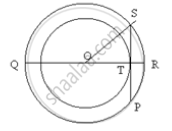Share

Books Shortlist

# Two Circle of Radii 5 Cm and 3 Cm Are Concentric. Calculate the Length of a Chord of the Outer Circle Which Touches the Inner - ICSE Class 10 - Mathematics

ConceptTangent Properties - If Two Circles Touch, the Point of Contact Lies on the Straight Line Joining Their Centers

#### Question

Two circle of radii 5 cm and 3 cm are concentric. Calculate the length of a chord of the outer circle which touches the inner

#### Solution\

OS = 5 cm
OT = 3 cm
In Rt. Triangle OST
By Pythagoras Theorem,
ST^2 =OS^2 - OT^2
 ST^2 =25-9
ST^2 =16
ST = 4cm

Since OT is perpendicular to SP and OT bisects chord SP
So, SP = 8 cm

Is there an error in this question or solution?

#### APPEARS IN

Solution Two Circle of Radii 5 Cm and 3 Cm Are Concentric. Calculate the Length of a Chord of the Outer Circle Which Touches the Inner Concept: Tangent Properties - If Two Circles Touch, the Point of Contact Lies on the Straight Line Joining Their Centers.
S